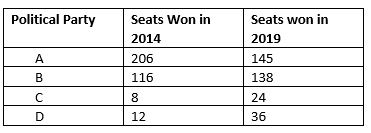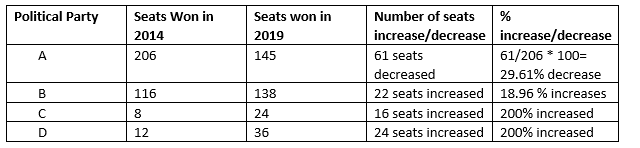# Extra questions for comparing quantities Class 8

In this page we have Comparing Quantities Class 8 extra questions with Answers . Hope you like them and do not forget to like , social share and comment at the end of the page.
Question 1
Out of a salary of Rs 75000, I kept 1/3 as savings. Out of the remaining money, I spend 25 % on food and 40 % on house rent. How much do I spend on food and house rent?

Money for Saving= 1/3 of Rs 75000 = Rs 25000
Money left= Rs 75000- Rs 25000= Rs 50,000
Money spent on food = 25% of Rs 50,000= Rs 12500
Money spent on house = 45% of Rs 50,000= Rs 20000

Question 2
A big mega store is having a festival sale and all the items are having 25% discount.
Vivek is having a Rs 50 Gift card and he has chosen a game whose marked price is Rs 300. Now Vivek is confused how to calculate the discount and what remaining money need to be paid to the clerk
The sales clerk has suggested two ways to calculate the concession
Method 1: Subtract Rs 50 from Marked price and then take 25% discount on the remaining amount
Method 2: Take 25% discount on the Marked price and then subtract Rs 50 from it
Now the questions for you
(a) Do you think both the method will give same result? If not predict which method will be beneficial to him
(b) Calculate the amount Vivek has to pay to the clerk in each case
(c) What method do the store actually uses? And why?

Lets first calculate using both the method
Method 1
MP = Rs 300
MP After Rs 50 deduction = Rs 300 -50=Rs 250
Discount = 25% of Rs 250 = Rs 62.5
Price after discount= Rs 250 - Rs 62.5= Rs 187.5
Method 2
MP = Rs 300
Discount = 25% of Rs 300 = Rs 75
Price after discount= Rs 300 - Rs 75= Rs 225
Amount left afte Rs 50 gift deduction = Rs 225 - 50 = Rs 175
So both the method gave different result. And method -2 is beneficial.
Method -2 is correct method and this is used by the stores

Question 3
Rina gets 94 marks in her exams. These are 47% of the total marks. Find the maximum number of marks.

Lets x be the maximum number of marks
94 = 47% of x
94= 47x/100
x= 200

Question 4
A tank can hold 50 liters of water. At present, it is only 30 % full. How many liters of water shall I pit in the tank, so that it is 50 % full?

Present status = 30% of 50 = 15 Litres
target status= 50% of 50 = 25 Litres
So water required = 25-15=10 L

Question 5
In case of gain on the selling of product.
Selling price is defined as
S. P= [1+ (Gain%/100)] × C.P
check if this is true

Gain = (Gain%/100) × C.P
SP= C.P + Gain= C.P + (Gain%/100) × C.P= [1+ (Gain%/100)] × C.P
So this statement is correct

Question 6
Fill in the blank
(a) Five times a number is a ______ % increase in the number
(b) The cost price of 10 cardboards is same as Selling price of 5 cardboards. The profit % in this transaction is ___________
(c) The discount on an item is calculated on the _________ of the item
(d)______ is charged on the sale of an item by government and is added to the bill amount

(a) 400%
(b) 100%
(c) Marked price
(d) Sales tax

Question 7
A's income is 20 % more than B. How much percent is B income less than A?

let x be B income
A income = x + 20% of x = 1.2x
Amount B's income less than A's =1.2x -x=.2x
% B income less than A = (.2x/1.2x) × 100=16.66%

Question 8
Find the following
(a) MP =Rs 1000, Discount 10%, SP=?
(b) SP =Rs 81, Discount 10%, MP =?
(c) MP=Rs 625, SP=Rs 562.50, Discount%=?

(a) Discount= 10% of 1000 Rs 100
SP= MP -discount= Rs 900
(b)let x be the MP
Discount =10% of x = 10x/100
SP = x - x/10= 9x/10
Now
9x/10=81
x=90
(c) Discount = MP -SP= 625 - 562.5=62.5
%= (62.5/625) × 100=10%

Question 9
Sachin bought a pair of Reebok shoes at a sale of 25%. If the amount he paid was Rs 2700, find the marked price.

let x be the MP
Discount =25% of x = x/4
SP = x - x/4= 3x/4
Now
3x/4=2700
x=Rs 3600

Question 10
In an election, there are a total of 60000 voters and there are two candidates, A and B. 60 % of the voters go for polls out of which 60 % vote for A. How any votes do B get?

Votes who went to poll=60% of 60000= 36000
Number of Votes A got = 60% of 36000=21600
Number of Votes B get= 36000-21600=14400

Question 11
If the Principal is Rs 50000 and 8% is the rate of interest per annum compound annually
(a) Find the interest earned in First year
(b) What is the principal for 2nd Year
(c)What is the interest earned in second year
(d) Is First year interest same as second year interest
(e) What will be the total interest earned for 2 years if it were simple interest per annum

(a) interest earned in First year
$I_1= \frac {50000 \times 8 \times 1}{100}=Rs \; 4000$
(b) principal for 2nd Year= Principle in Ist year + interest earned = Rs 50000 + Rs 4000= Rs 54000
(c)interest earned in second year
$I_2= \frac {54000 \times 8 \times 1}{100}=Rs \; 4320$
(d) Interest are different
(e) total interest earned for 2 years if it were simple interest per annum
$I=\frac {50000 \times 8 \times 2}{100}=Rs \; 8000$

Question 12
An agent of an insurance company gets a commission of 8 % on the basic premium he collects. What will be the commission earned by him if he collects Rs 4800?

Commision = 8% of Rs 4800=Rs 384

Question 13
There are 400 boy students in class X in all the section in Saint Xavier school. If 25% of the students are girls, find the total number of students in class X

Number of Boys= 75% of 400= 300

Question 14
You buy a house for Rs 400,0000 and pay a tax of 8%. How much is tax?

Tax= 8% of Rs 400,0000 = Rs 320,000

Question 15
Z% of 100 + 10% of 50 = 100
Find the value of z

Z% of 100 + 10% of 50 = 100

z + .5 = 100
z= 99.5

Question 16
What is the percentage increase or decrease in the number of seats won by A, B, C and D in the general elections of 2019 as compared to the results of 2014?## Summary

This Comparing Quantities Class 8 extra questions with Answers is prepared keeping in mind the latest syllabus of CBSE . This has been designed in a way to improve the academic performance of the students. If you find mistakes , please do provide the feedback on the mail. You can download this as PDF also